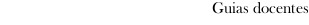Educational guide 2015_16Escola de Enxeñaría Industrial
 Grao en Enxeñaría MecánicaSubjectsSystems for Data Analysis, Simulation and ValidationContents
 Topic Sub-topic Presentation of the matter - Introduction to the matter- previous Knowledges: design of machines; software of *modelado,analysis, simulation and validation- Definition of the project to realise: design, analysis, simulation andvalidation of a machine Calculation of axes and trees - Definition of the element- theoretical Calculation and selection- Software of calculation Calculation of gears - Definition of the element- theoretical Calculation and selection- Software of calculation Calculation of rollings and *cojinetes - Definition of the element- theoretical Calculation and selection- Software of calculation Calculation of unions:- unions axis-cube and tolerances- unions soldered and hit- unions *atornilladas and *roblonadas - Definition of the element- theoretical Calculation and selection- Software of calculation Calculation of *resortes - Definition of the element- theoretical Calculation and selection- Software of calculation Calculation of belts and chains - Definition of the element- theoretical Calculation and selection- Software of calculation
 Universidade de Vigo            | Reitoría | Campus Universitario | C.P. 36.310 Vigo (Pontevedra) | Spain | Tlf: +34 986 812 000